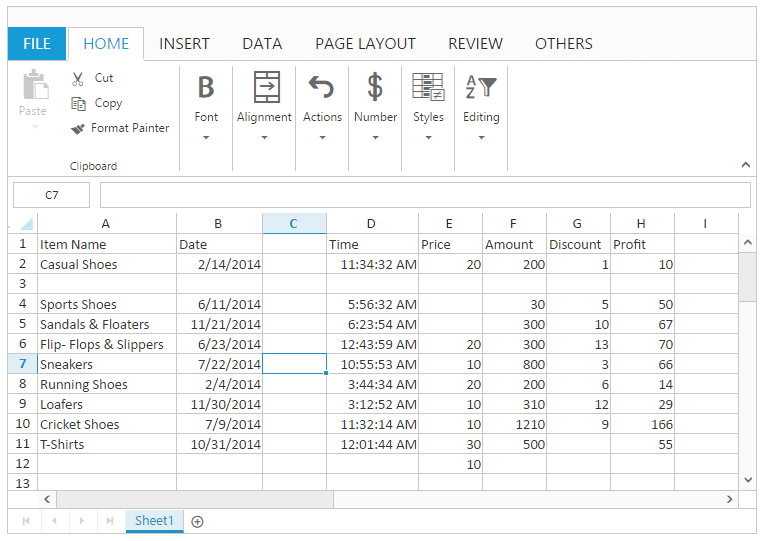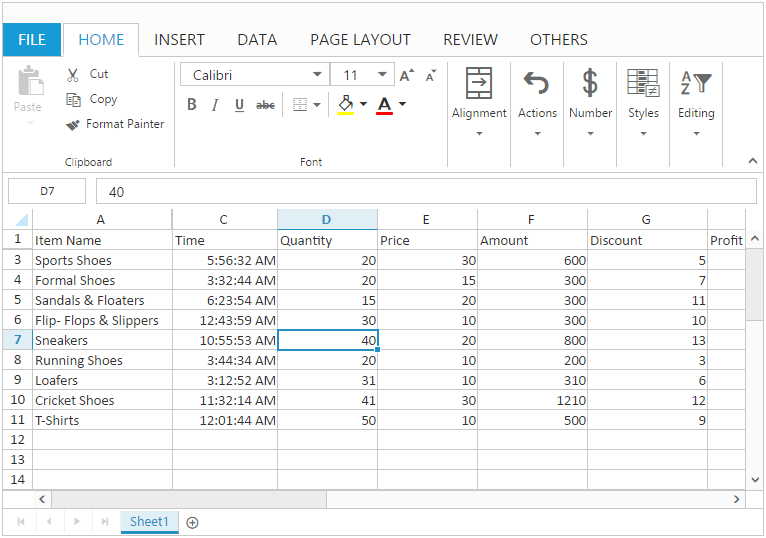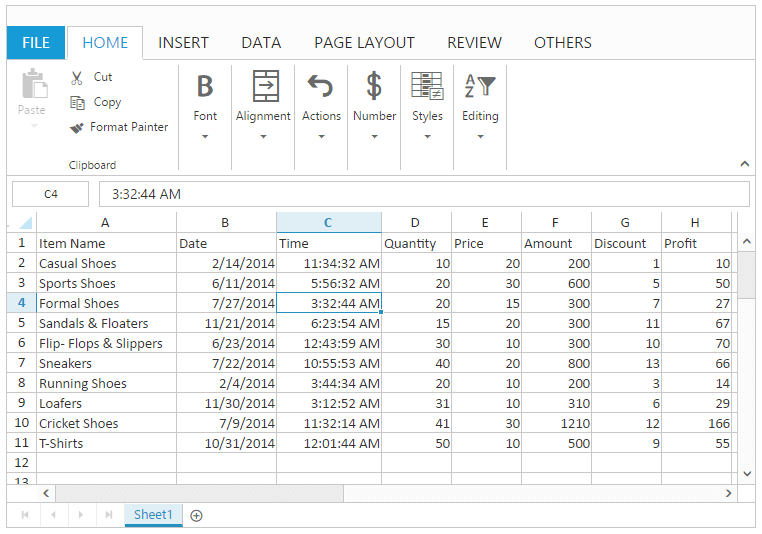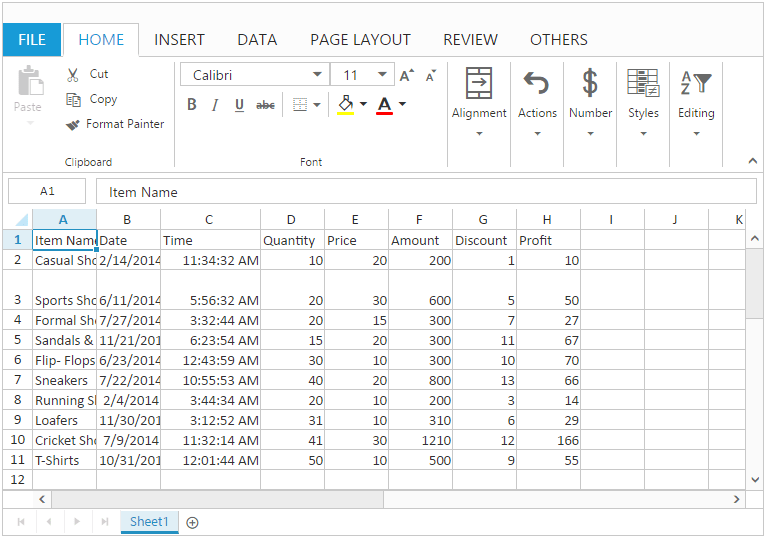# Rows and Columns

28 Feb 201724 minutes to read

Spreadsheet is a tabular format consisting of rows and columns. Rows and columns are used to represent the editing area in Spreadsheet. The intersection point of rows and columns are called as cells. In that you can perform editing. You have `row-count` and `col-count` in sheets property for defining the rows and columns count. By default, Spreadsheet creates `20` rows and `21` columns. Based on this grid content will be created.

## Rows

Rows are a collection of cells that run horizontally. Each row is identified by the row number in the row header.

## Columns

Columns are a collection of cells that run vertically. Each column is identified by column heading in the column header.

The following code example describes the above behavior.

``````<ej-spread-sheet id="Spreadsheet">
<e-sheets>
<e-sheet row-count="50" col-count="36">
<e-range-settings>
<e-range-setting datasource="ViewBag.Datasource" ></e-range-setting>
</e-range-settings>
</e-sheet>
</e-sheets>
``````namespace MVCSampleBrowser.Controllers
{
public partial class SpreadsheetController : Controller
{
public ActionResult Default()
{
List<ItemDetail> lItems = new List<ItemDetail>();
lItems.Add(new ItemDetail() { ItemName = "Casual Shoes", Date = new DateTime(2014, 02, 14), Time = new DateTime(2014, 02, 14, 11, 34, 32), Quantity = 10, Price = 20, Amount = 200, Discount = 1, Profit = 10 });
lItems.Add(new ItemDetail() { ItemName = "Sports Shoes", Date = new DateTime(2014, 06, 11), Time = new DateTime(2014, 06, 11, 05, 56, 32), Quantity = 20, Price = 30, Amount = 600, Discount = 5, Profit = 50 });
lItems.Add(new ItemDetail() { ItemName = "Formal Shoes", Date = new DateTime(2014, 07, 27), Time = new DateTime(2014, 07, 27, 03, 32, 44), Quantity = 20, Price = 15, Amount = 300, Discount = 7, Profit = 27 });
lItems.Add(new ItemDetail() { ItemName = "Sandals & Floaters", Date = new DateTime(2014, 11, 21), Time = new DateTime(2014, 11, 21, 06, 23, 54), Quantity = 15, Price = 20, Amount = 300, Discount = 11, Profit = 67 });
lItems.Add(new ItemDetail() { ItemName = "Flip- Flops & Slippers", Date = new DateTime(2014, 06, 23), Time = new DateTime(2014, 06, 23, 12, 43, 59), Quantity = 30, Price = 10, Amount = 300, Discount = 10, Profit = 70 });
ViewBag.Datasource = lItems;
return View();
}
}
}``````

## List of operations

You can perform the following list of operations in rows and columns,

• Insert
• Delete
• Show and Hide
• Resizing

## Insert

You can insert blank cells, rows or columns based on the selection in a worksheet. You have to enable the `allow-insert` property to perform the insert operation. You can perform insert operation through,

• OTHERS tab in ribbon.

NOTE

In the header context menu you can insert only rows or columns.

### Insert Shift Bottom

You can dynamically insert blank cells to the top of the selected range and shift the selected cells to down by following ways,

• Click Insert in the context menu and select “Shift Cells Down” option in Insert dialog.
• Select Insert Cells option in Insert button of OTHERS tab in Ribbon and select “Shift Cells Down” option in Insert dialog.

You can also perform insert shift bottom using `insertShiftBottom` method.

### Insert Shift Right

You can dynamically insert blank cells to the left of the selected range and shift the selected cells to right by following ways,

• Click Insert in the context menu and select “Shift Cells Right” option in Insert dialog.
• Select Insert Cells option in Insert button of OTHERS tab in Ribbon and select “Shift Cells Right” option in Insert dialog.

You can also perform insert shift right using `insertShiftRight` method.

### Insert Entire Row

You can dynamically insert the selected number of blank rows to the top of the selected range by following ways,

• Click Insert in the context menu and select “Entire Row” option in Insert dialog.
• Select Insert Cells option in Insert button of OTHERS tab in Ribbon and select “Entire Row” option in Insert dialog.
• Select Insert Sheet Rows option in Insert button of OTHERS tab in Ribbon.

You can also perform insert entire row using `insertEntireRow` method.

### Insert Entire Column

You can dynamically insert the selected number of blank columns to the left of the selected range by following ways,

• Click Insert in the context menu and select “Entire Column” option in Insert dialog.
• Select Insert Cells option in Insert button of OTHERS tab in Ribbon and select “Entire Column” option in Insert dialog.
• Select Insert Sheet Columns option in Insert button of OTHERS tab in Ribbon.

You can also perform insert entire column using `insertEntireColumn` method.

## Delete

You can delete a range of cells, rows or columns based on the selection in worksheet. You have to enable the `allow-delete` property to perform delete operation.
You can perform delete operation through,

• OTHERS tab in Ribbon

NOTE

### Delete Shift Up

You can dynamically delete the selected range of cells and shift the other cells to top by following ways,

• Click Delete in the context menu and select “Shift Cells Up” option in Delete dialog.
• Select Delete Cells option in Delete button of OTHERS tab in Ribbon and select “Shift Cells Up” option in Delete dialog.

You can also perform delete shift up using `deleteShiftUp` method.

### Delete Shift Left

You can dynamically delete the selected range of cells and shift the other cells to left by following ways,

• Click Delete in the context menu and select “Shift Cells Left” option in Delete dialog.
• Select Delete Cells in Delete button of OTHERS tab in Ribbon and select “Shift Cells Left” option in Delete dialog.

You can also perform delete shift left using `deleteShiftLeft` method.

### Delete Entire Row

You can dynamically delete the selected rows and shift the other rows to top by following ways,

• Click Delete in the context menu and select “Entire Row” option in Delete dialog.
• Select Delete Cells option in Delete button of OTHERS tab in Ribbon and select “Entire Row” option in Delete dialog.
• Select Delete Sheet Rows option in Delete button of OTHERS tab in Ribbon.

You can also perform delete entire row using `deleteEntireRow` method.

### Delete Entire Column

You can dynamically delete a selected columns and shift other columns to left by following ways,

• Click Delete in the context menu and select “Entire Column” option in Delete dialog.
• Select Delete Cells option in Delete button of OTHERS tab in Ribbon and select “Entire Column” option in Delete dialog.
• Select Delete Sheet Columns option in Delete button of OTHERS tab in Ribbon.

You can also perform delete entire column using `deleteEntireColumn` method.

The following code example describes the above behavior.

``````<ej-spread-sheet id="Spreadsheet" load-complete="loadComplete">
<e-sheets>
<e-sheet>
<e-range-settings>
<e-range-setting datasource="ViewBag.Datasource" ></e-range-setting>
</e-range-settings>
</e-sheet>
</e-sheets>

<script type="text/javascript">
if (!this.isImport) {
this.insertEntireRow(2, 2);
this.insertEntireColumn(2, 2);
this.deleteEntireRow(4, 4);
this.deleteEntireColumn(4, 4);
this.insertShiftBottom({ rowIndex: 4, colIndex: 4 }, { rowIndex: 4, colIndex: 4 });
this.insertShiftRight({ rowIndex: 3, colIndex: 4 }, { rowIndex: 3, colIndex: 4 });
this.deleteShiftUp({ rowIndex: 4, colIndex: 6 }, { rowIndex: 4, colIndex: 6 });
this.deleteShiftLeft({ rowIndex: 3, colIndex: 6 }, { rowIndex: 3, colIndex: 6 });
}
}
</script>``````
``````namespace MVCSampleBrowser.Controllers
{
public partial class SpreadsheetController : Controller
{
public ActionResult Default()
{
List<ItemDetail> lItems = new List<ItemDetail>();
lItems.Add(new ItemDetail() { ItemName = "Casual Shoes", Date = new DateTime(2014, 02, 14), Time = new DateTime(2014, 02, 14, 11, 34, 32), Quantity = 10, Price = 20, Amount = 200, Discount = 1, Profit = 10 });
lItems.Add(new ItemDetail() { ItemName = "Sports Shoes", Date = new DateTime(2014, 06, 11), Time = new DateTime(2014, 06, 11, 05, 56, 32), Quantity = 20, Price = 30, Amount = 600, Discount = 5, Profit = 50 });
lItems.Add(new ItemDetail() { ItemName = "Formal Shoes", Date = new DateTime(2014, 07, 27), Time = new DateTime(2014, 07, 27, 03, 32, 44), Quantity = 20, Price = 15, Amount = 300, Discount = 7, Profit = 27 });
lItems.Add(new ItemDetail() { ItemName = "Sandals & Floaters", Date = new DateTime(2014, 11, 21), Time = new DateTime(2014, 11, 21, 06, 23, 54), Quantity = 15, Price = 20, Amount = 300, Discount = 11, Profit = 67 });
lItems.Add(new ItemDetail() { ItemName = "Flip- Flops & Slippers", Date = new DateTime(2014, 06, 23), Time = new DateTime(2014, 06, 23, 12, 43, 59), Quantity = 30, Price = 10, Amount = 300, Discount = 10, Profit = 70 });
ViewBag.Datasource = lItems;
return View();
}
}
}``````

The following output is displayed as a result of the above code example.## Show and Hide

You can show or hide the rows and columns in Spreadsheet using methods and context menu.

### Hide Row

You can hide the rows dynamically by using one of the following ways,

• Hide the rows using `hideRow` method.

### Hide Column

You can hide the columns dynamically by using one of the following ways,

The following code example describes the above behavior.

``````<ej-spread-sheet id="Spreadsheet" load-complete="loadComplete">
<e-sheets>
<e-sheet>
<e-range-settings>
<e-range-setting datasource="ViewBag.Datasource" ></e-range-setting>
</e-range-settings>
</e-sheet>
</e-sheets>

<script type="text/javascript">
if (!this.isImport) {
this.hideRow(2);
this.hideColumn(2);
}
}
</script>``````
``````namespace MVCSampleBrowser.Controllers
{
public partial class SpreadsheetController : Controller
{
public ActionResult Default()
{
List<ItemDetail> lItems = new List<ItemDetail>();
lItems.Add(new ItemDetail() { ItemName = "Casual Shoes", Date = new DateTime(2014, 02, 14), Time = new DateTime(2014, 02, 14, 11, 34, 32), Quantity = 10, Price = 20, Amount = 200, Discount = 1, Profit = 10 });
lItems.Add(new ItemDetail() { ItemName = "Sports Shoes", Date = new DateTime(2014, 06, 11), Time = new DateTime(2014, 06, 11, 05, 56, 32), Quantity = 20, Price = 30, Amount = 600, Discount = 5, Profit = 50 });
lItems.Add(new ItemDetail() { ItemName = "Formal Shoes", Date = new DateTime(2014, 07, 27), Time = new DateTime(2014, 07, 27, 03, 32, 44), Quantity = 20, Price = 15, Amount = 300, Discount = 7, Profit = 27 });
lItems.Add(new ItemDetail() { ItemName = "Sandals & Floaters", Date = new DateTime(2014, 11, 21), Time = new DateTime(2014, 11, 21, 06, 23, 54), Quantity = 15, Price = 20, Amount = 300, Discount = 11, Profit = 67 });
lItems.Add(new ItemDetail() { ItemName = "Flip- Flops & Slippers", Date = new DateTime(2014, 06, 23), Time = new DateTime(2014, 06, 23, 12, 43, 59), Quantity = 30, Price = 10, Amount = 300, Discount = 10, Profit = 70 });
ViewBag.Datasource = lItems;
return View();
}

}
}``````

The following output is displayed as a result of the above code example.### Show Row

You can show the hidden rows dynamically by using one of the following ways,

• Show the hidden rows using `showRow` method.

### Show Column

You can show the hidden columns dynamically by using one of the following ways,

• Show the hidden columns using `showColumn` method.

The following code example describes the above behavior.

``````<ej-spread-sheet id="Spreadsheet" load-complete="loadComplete">
<e-sheets>
<e-sheet>
<e-range-settings>
<e-range-setting datasource="ViewBag.Datasource" ></e-range-setting>
</e-range-settings>
</e-sheet>
</e-sheets>

<script type="text/javascript">
if (!this.isImport) {
this.hideRow(2);
this.hideColumn(2);
this.showRow(2);
this.showColumn(2);
}
}
</script>``````
``````namespace MVCSampleBrowser.Controllers
{
public partial class SpreadsheetController : Controller
{
public ActionResult Default()
{
List<ItemDetail> lItems = new List<ItemDetail>();
lItems.Add(new ItemDetail() { ItemName = "Casual Shoes", Date = new DateTime(2014, 02, 14), Time = new DateTime(2014, 02, 14, 11, 34, 32), Quantity = 10, Price = 20, Amount = 200, Discount = 1, Profit = 10 });
lItems.Add(new ItemDetail() { ItemName = "Sports Shoes", Date = new DateTime(2014, 06, 11), Time = new DateTime(2014, 06, 11, 05, 56, 32), Quantity = 20, Price = 30, Amount = 600, Discount = 5, Profit = 50 });
lItems.Add(new ItemDetail() { ItemName = "Formal Shoes", Date = new DateTime(2014, 07, 27), Time = new DateTime(2014, 07, 27, 03, 32, 44), Quantity = 20, Price = 15, Amount = 300, Discount = 7, Profit = 27 });
lItems.Add(new ItemDetail() { ItemName = "Sandals & Floaters", Date = new DateTime(2014, 11, 21), Time = new DateTime(2014, 11, 21, 06, 23, 54), Quantity = 15, Price = 20, Amount = 300, Discount = 11, Profit = 67 });
lItems.Add(new ItemDetail() { ItemName = "Flip- Flops & Slippers", Date = new DateTime(2014, 06, 23), Time = new DateTime(2014, 06, 23, 12, 43, 59), Quantity = 30, Price = 10, Amount = 300, Discount = 10, Profit = 70 });
ViewBag.Datasource = lItems;
return View();
}

}
}``````

The following output is displayed as a result of the above code example.## Resizing

You can change `column-width` and `row-height` with the specified value. You have to enable `allow-resizing` property to perform resizing.

You can perform resizing using one of the following ways,

• Set the column width by using `setColWidth` method or `column-width` property.
• Set the row height by using `setRowHeight` method or `row-height` property.

The following code example describes the above behavior.

``````<ej-spread-sheet id="Spreadsheet" load-complete="loadComplete">
<e-sheets>
<e-sheet row-height="20" column-width="64">
<e-range-settings>
<e-range-setting datasource="ViewBag.Datasource" ></e-range-setting>
</e-range-settings>
</e-sheet>
</e-sheets>

<script type="text/javascript">
if (!this.isImport) {
this.XLResize.setColWidth(2, 100);
this.XLResize.setRowHeight(2, 40);
}
}
</script>``````
``````namespace MVCSampleBrowser.Controllers
{
public partial class SpreadsheetController : Controller
{
public ActionResult Default()
{
List<ItemDetail> lItems = new List<ItemDetail>();
lItems.Add(new ItemDetail() { ItemName = "Casual Shoes", Date = new DateTime(2014, 02, 14), Time = new DateTime(2014, 02, 14, 11, 34, 32), Quantity = 10, Price = 20, Amount = 200, Discount = 1, Profit = 10 });
lItems.Add(new ItemDetail() { ItemName = "Sports Shoes", Date = new DateTime(2014, 06, 11), Time = new DateTime(2014, 06, 11, 05, 56, 32), Quantity = 20, Price = 30, Amount = 600, Discount = 5, Profit = 50 });
lItems.Add(new ItemDetail() { ItemName = "Formal Shoes", Date = new DateTime(2014, 07, 27), Time = new DateTime(2014, 07, 27, 03, 32, 44), Quantity = 20, Price = 15, Amount = 300, Discount = 7, Profit = 27 });
lItems.Add(new ItemDetail() { ItemName = "Sandals & Floaters", Date = new DateTime(2014, 11, 21), Time = new DateTime(2014, 11, 21, 06, 23, 54), Quantity = 15, Price = 20, Amount = 300, Discount = 11, Profit = 67 });
lItems.Add(new ItemDetail() { ItemName = "Flip- Flops & Slippers", Date = new DateTime(2014, 06, 23), Time = new DateTime(2014, 06, 23, 12, 43, 59), Quantity = 30, Price = 10, Amount = 300, Discount = 10, Profit = 70 });
ViewBag.Datasource = lItems;
return View();
}

}
}``````

The following output is displayed as a result of the above code example.